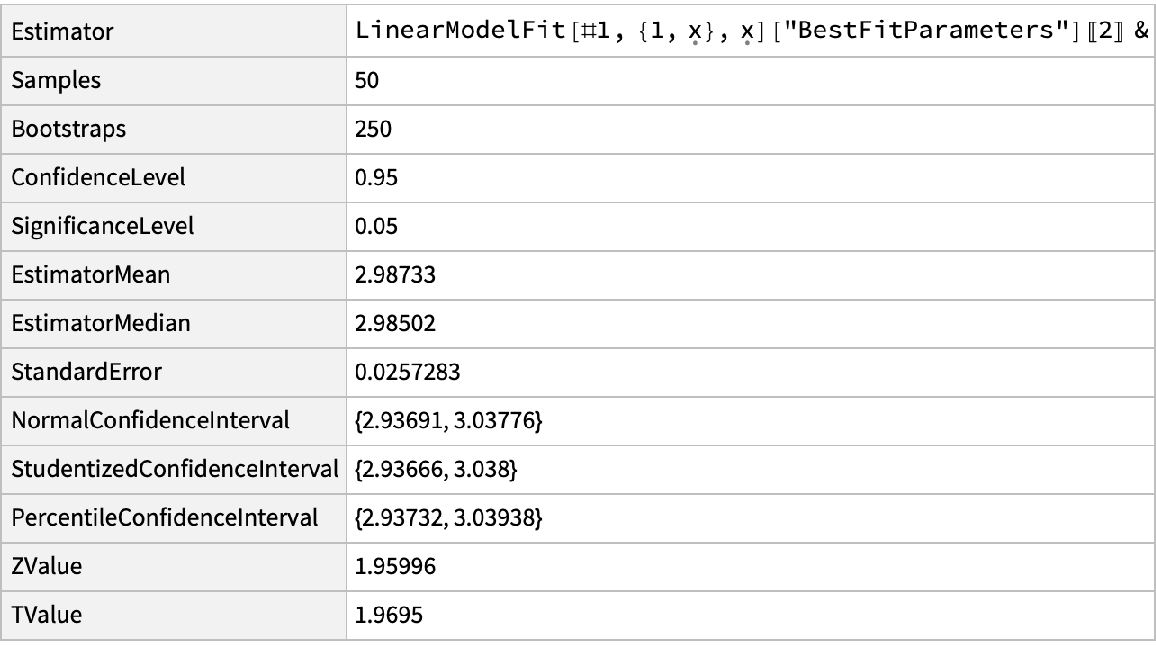#Function Repository Resource:

# BootstrappedEstimate

Perform bootstrapping of an estimator on some data

Contributed by: Sander Huisman
 ResourceFunction["BootstrappedEstimate"][data,e,n] bootstraps the data data using the estimator e with n bootstrap samples.

## Details and Options

Bootstrapping involves randomly sampling elements from a dataset and calculating the estimator multiple times. The variance in the found estimator is then an indicator for the uncertainty of the estimator.
For each run (out of n), the estimator e is calculated for a randomly sampled set of elements from data (with possible repeats). This will result in n estimates for the estimator e. From these, the standard deviation is calculated, which is then interpreted as a standard error. Based on the confidence level, the various intervals are then calculated. Based on the distribution of these estimates, we can calculate the quantiles for a given confidence interval ("PercentileConfidenceInterval"). This will take into account the actual distribution of the underlying estimates. We can also assume this is a normal distribution, and from the CDF of the normal distribution, the number of σ away from the mean is calculated to get the right confidence interval for a certain confidence level ("NormalConfidenceInterval"). If the number of samples is small, a correction can be used based on a Student T distribution ("StudentizedConfidenceInterval").
ResourceFunction["BootstrappedEstimate"] takes the following options:
 confidence level to use for confidence intervals significance level to use for confidence intervals "Samples" number of samples in each bootstrap
The default ConfidenceLevel is 0.95 (95%, corresponding to a SignificanceLevel of 5%).
The default value for the option "Samples" is the length of the original data.
If both ConfidenceLevel and SignificanceLevel options are given, the value for ConfidenceLevel will be used.

## Examples

### Basic Examples (1)

Bootstrap the mean of a randomly created dataset:

 In:=Out=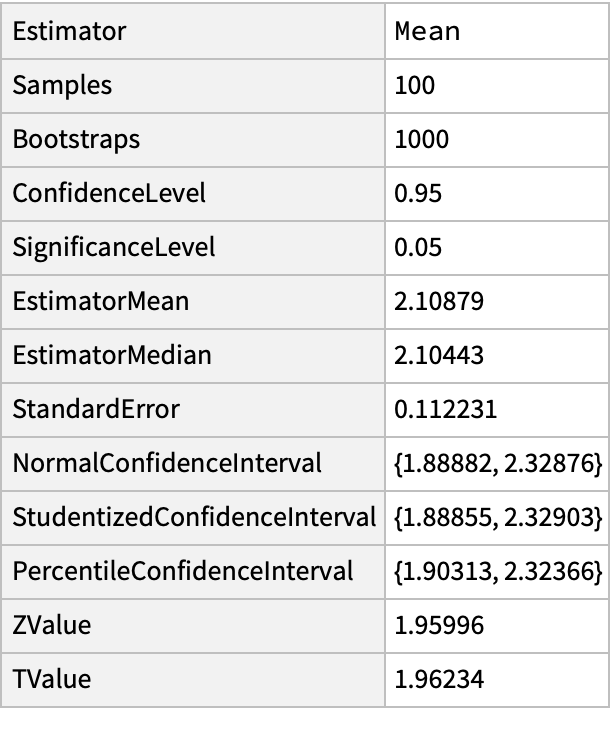### Options (3)

#### ConfidenceLevel (1)

Find the kurtosis and report back the confidence interval corresponding to a 99% confidence level:

 In:=Out=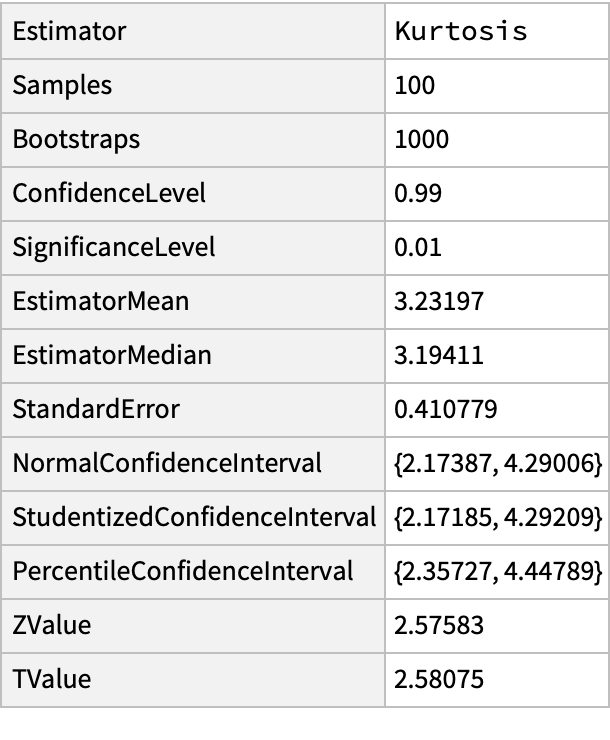#### SignificanceLevel (1)

Find the skewness for a 1% significance level:

 In:=Out=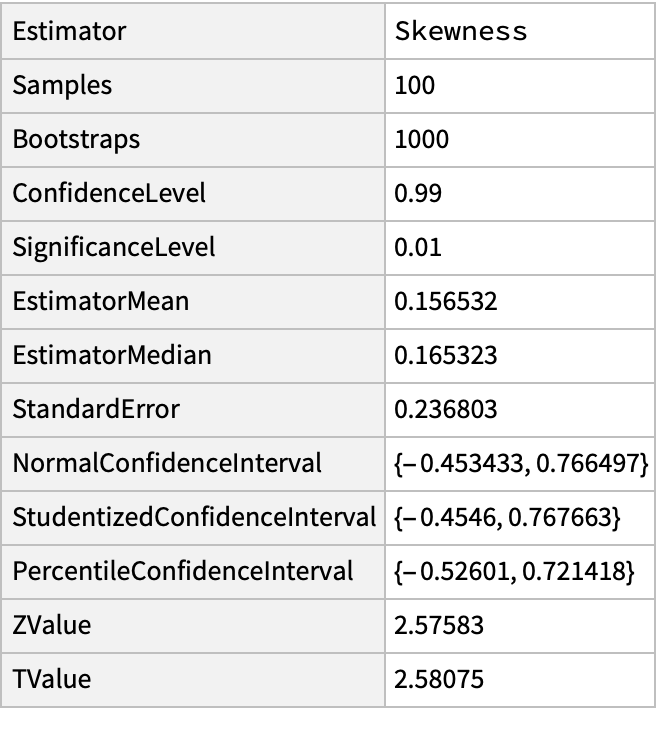#### Samples (1)

Use a higher number of samples for each bootstrap:

 In:=Out=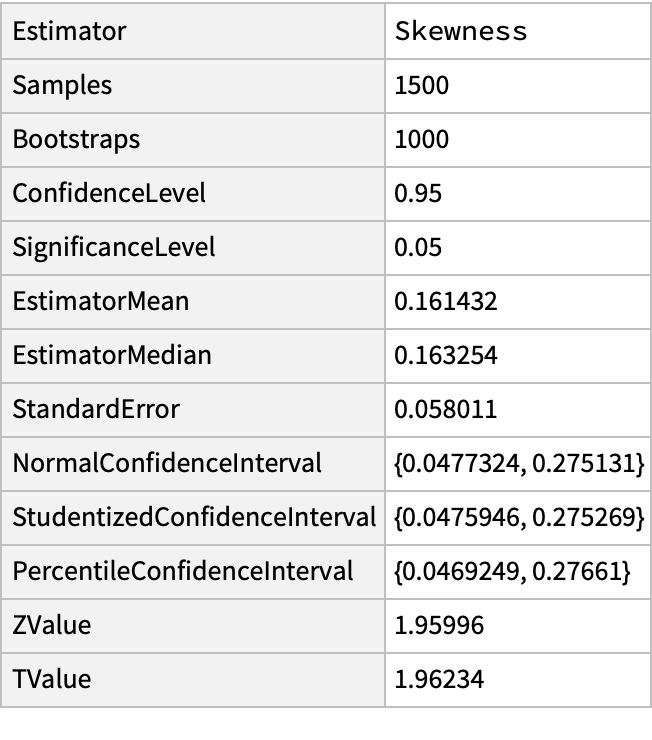### Neat Examples (2)

Create a random dataset:

 In:=Out=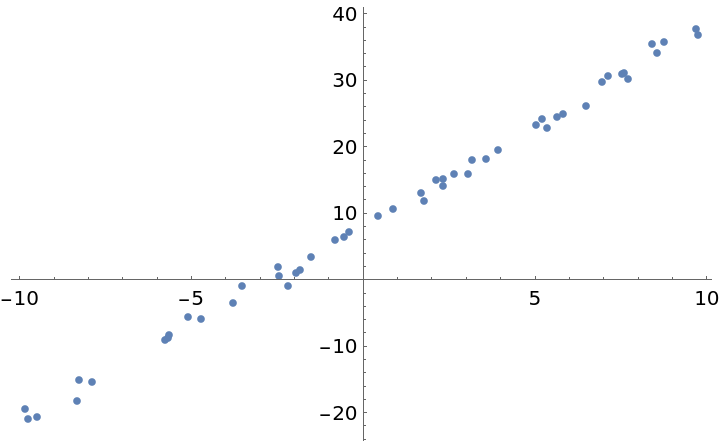Perform bootstrapping on the slope by doing repeated linear fitting:

 In:=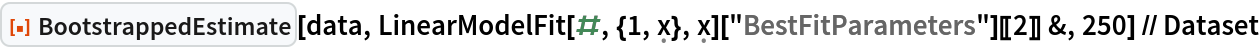Out=